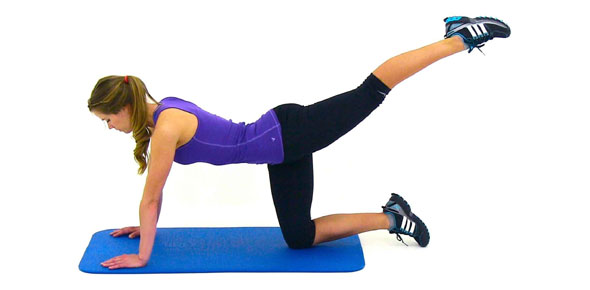# Science Exercises For PMR

5 QuestionsSettingsRelated Topics
• 1.
The following are the steps to use a Bunsen burner. J - Turn on the glass slowly K - Hold a lighted match or wooden splinter near the mouth of the barrel. L -  Close the air hole completely by turning the collar. M - When the flame is seen, slowly open the air hole by turning the collar.
• A.

J, L, M, K

• B.

L, K, J, M

• C.

K, J, L, M

• D.

M, L, K, J

• 2.
When two objects of different temperatures are brought together,
• A.

The heat in both objects increase.

• B.

Heat is transfered from the warmer object to the cooler object.

• C.

Both objects become cooler.

• D.

The temperature of both objects remains the same.

• 3.
Given the density of iron is 6 000kg m-3 , calculate the mass of an iron box measuring 10m x 2m x 2m.
• A.

8 000kg

• B.

16 000kg

• C.

32 000kg

• D.

240 000kg

• 4.
Which of the following is an example of unicellular organism?
• A.

Mushroom

• B.

Paramecium

• C.

Spirogyra

• D.

Mucor

• 5.
Which  of the following statements are incorrect?
• A.

Heat is how hot an object is

• B.

Heat is measured in joules

• C.

Heat is an energy

• D.

Heat can be transferred